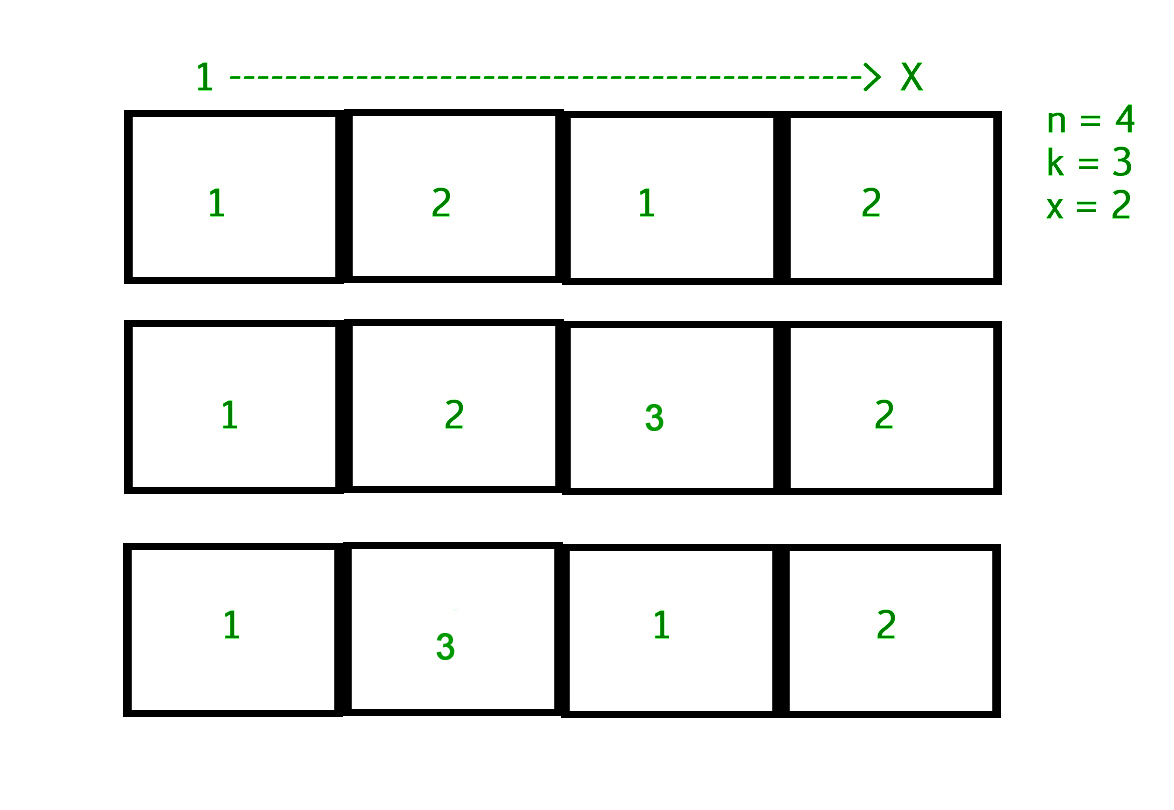Related Articles
Count of arrays having consecutive element with different values
• Difficulty Level : Hard
• Last Updated : 21 Apr, 2021

Given three positive integers n, k and x. The task is to count the number of different array that can be formed of size n such that each element is between 1 to k and two consecutive element are different. Also, the first and last elements of each array should be 1 and x respectively.

Examples :

```Input : n = 4, k = 3, x = 2
Output : 3```The idea is to use Dynamic Programming and combinatorics to solve the problem.
First of all, notice that the answer is same for all x from 2 to k. It can easily be proved. This will be useful later on.
Let the state f(i) denote the number of ways to fill the range [1, i] of array A such that A1 = 1 and Ai ≠ 1.
Therefore, if x ≠ 1, the answer to the problem is f(n)/(k – 1), because f(n) is the number of way where An is filled with a number from 2 to k, and the answer are equal for all such values An, so the answer for an individual value is f(n)/(k – 1).
Otherwise, if x = 1, the answer is f(n – 1), because An – 1 ≠ 1, and the only number we can fill An with is x = 1.

Now, the main problem is how to calculate f(i). Consider all numbers that Ai – 1 can be. We know that it must lie in [1, k].

• If Ai – 1 ≠ 1, then there are (k – 2)f(i – 1) ways to fill in the rest of the array, because Ai cannot be 1 or Ai – 1 (so we multiply with (k – 2)), and for the range [1, i – 1], there are, recursively, f(i – 1) ways.
• If Ai – 1 = 1, then there are (k – 1)f(i – 2) ways to fill in the rest of the array, because Ai – 1 = 1 means Ai – 2 ≠ 1 which means there are f(i – 2)ways to fill in the range [1, i – 2] and the only value that Ai cannot be 1, so we have (k – 1) choices for Ai

By combining the above, we get

`f(i) = (k - 1) * f(i - 2) + (k - 2) * f(i - 1)`

This will help us to use dynamic programming using f(i).

Below is the implementation of this approach:

## C++

 `// CPP Program to find count of arrays.``#include ``#define MAXN 109``using` `namespace` `std;` `// Return the number of arrays with given constartints.``int` `countarray(``int` `n, ``int` `k, ``int` `x)``{``    ``int` `dp[MAXN] = { 0 };` `    ``// Initalising dp and dp.``    ``dp = 0;``    ``dp = 1;` `    ``// Computing f(i) for each 2 <= i <= n.``    ``for` `(``int` `i = 2; i < n; i++)``        ``dp[i] = (k - 2) * dp[i - 1] +``                ``(k - 1) * dp[i - 2];` `    ``return` `(x == 1 ? (k - 1) * dp[n - 2] : dp[n - 1]);``}` `// Driven Program``int` `main()``{``    ``int` `n = 4, k = 3, x = 2;``    ``cout << countarray(n, k, x) << endl;``    ``return` `0;``}`

## Java

 `// Java program to find count of arrays.``import` `java.util.*;` `class` `Counting``{``    ``static` `int` `MAXN = ``109``;` `    ``public` `static` `int` `countarray(``int` `n, ``int` `k,``                                       ``int` `x)``    ``{``        ``int``[] dp = ``new` `int``[``109``];` `        ``// Initalising dp and dp.``        ``dp[``0``] = ``0``;``        ``dp[``1``] = ``1``;` `        ``// Computing f(i) for each 2 <= i <= n.``        ``for` `(``int` `i = ``2``; i < n; i++)``            ``dp[i] = (k - ``2``) * dp[i - ``1``] +``                ``(k - ``1``) * dp[i - ``2``];` `        ``return` `(x == ``1` `? (k - ``1``) * dp[n - ``2``] :``                                  ``dp[n - ``1``]);``    ``}``    ` `    ``// driver code``    ``public` `static` `void` `main(String[] args)``    ``{``        ``int` `n = ``4``, k = ``3``, x = ``2``;``        ``System.out.println(countarray(n, k, x));``    ``}``}`  `// This code is contributed by rishabh_jain`

## Python3

 `# Python3 code to find count of arrays.` `# Return the number of lists with``# given constraints.``def` `countarray( n , k , x ):``    ` `    ``dp ``=` `list``()``    ` `    ``# Initalising dp and dp``    ``dp.append(``0``)``    ``dp.append(``1``)``    ` `    ``# Computing f(i) for each 2 <= i <= n.``    ``i ``=` `2``    ``while` `i < n:``        ``dp.append( (k ``-` `2``) ``*` `dp[i ``-` `1``] ``+``                   ``(k ``-` `1``) ``*` `dp[i ``-` `2``])``        ``i ``=` `i ``+` `1``    ` `    ``return` `( (k ``-` `1``) ``*` `dp[n ``-` `2``] ``if` `x ``=``=` `1` `else` `dp[n ``-` `1``])` `# Driven code``n ``=` `4``k ``=` `3``x ``=` `2``print``(countarray(n, k, x))` `# This code is contributed by "Sharad_Bhardwaj".`

## C#

 `// C# program to find count of arrays.``using` `System;` `class` `GFG``{``// static int MAXN = 109;` `    ``public` `static` `int` `countarray(``int` `n, ``int` `k,``                                    ``int` `x)``    ``{``        ``int``[] dp = ``new` `int``;` `        ``// Initalising dp and dp.``        ``dp = 0;``        ``dp = 1;` `        ``// Computing f(i) for each 2 <= i <= n.``        ``for` `(``int` `i = 2; i < n; i++)``            ``dp[i] = (k - 2) * dp[i - 1] +``                    ``(k - 1) * dp[i - 2];` `        ``return` `(x == 1 ? (k - 1) * dp[n - 2] :``                                   ``dp[n - 1]);``    ``}``    ` `    ``// Driver code``    ``public` `static` `void` `Main()``    ``{``        ``int` `n = 4, k = 3, x = 2;``        ``Console.WriteLine(countarray(n, k, x));``    ``}``}`  `// This code is contributed by vt_m`

## PHP

 ``

## Javascript

 ``

Output :

`3`

My Personal Notes arrow_drop_up